# Logical Reasoning for Placement Test - Set 4

1)   In the following question, choose the correct code form.

The number/word group in the question is to be codified according to the following letter codes:

 Number 9 8 7 6 5 4 3 2 1 0 Letter Q U I C K L Y R O D

ROCKILY

a. 2165473
b. 2165347
c. 2156743
d. 2165743
 Answer  Explanation ANSWER: 2165743 Explanation: No explanation is available for this question!

2)   In the following question, choose the correct code form.

The number/word group in the question is to be codified according to the following letter codes:
 Number 9 8 7 6 5 4 3 2 1 0 Letter Q U I C K L Y R O D

3060589404

a. YDCDKUQLDL
b. YDCDKQULDL
c. YDCDKULQDL
d. YDCDKUDLQL
 Answer  Explanation ANSWER: YDCDKUQLDL Explanation: No explanation is available for this question!

3)   Directions :
1. There are 16 teams divided into 2 pools of 8 each.
2. Each team in a group plays against one another on a round-robin basis.
3. The top four teams from each group will qualify for the next round i.e. round 2.
4. In case of teams having the same number of wins, the team with better run-rate would be ranked ahead.
5. Draws are not allowed.

What is the minimum number of wins required to qualify for the next round?

a. 1
b. 2
c. 3
d. 4
 Answer  Explanation ANSWER: 2 Explanation: Each group consists of 8 teams. So, each team will play 7 matches each. Suppose each of the 8 teams were seeded and we consider the case where a higher seeded team will always win. So, the number of wins for the 8 teams would be 7, 6, 5, 4, 3, 2, 1, 0 with highest seeded team winning all and lowest seeded team losing all. For minimum number of wins we allow 3 teams to win maximum number of matches. Of the remaining 5 teams just find out the mean of their number of wins. In this case, it would be - (4+3+2+1+0)/5=2. So, 5 teams can end up with 2 wins each and a team with better run rate will qualify with 2 wins.The minimum number of wins required to qualify for next round with better run-rate is 2.

4)   Choose the word which is least like the other words in the group.

a. Chameleon
b. Crocodile
c. Snake
d. Locust
e. Skink
 Answer  Explanation ANSWER: Locust Explanation: Locust is an insect, while all other are reptiles.

5)   Choose the word which is not similar to the other words in the group.

a. Car
b. Cycle
c. Scooter
d. Helicopter
e. Airplane
 Answer  Explanation ANSWER: Cycle Explanation: All others run on fuel except cycle.

1. Antman, Batman, Cyclops, Deadpool, Electro, Falcon, and Gambit are standing near a wall and all of them are facing east.
2. Cyclops is on the immediate right of Deadpool.
3. Batman is at an extreme end and has Electro as his neighbor.
4. Gambit is between Electro and Falcon.
5. Deadpool is standing third from the south end.

Who is standing to the left of Electro?

a. Antman
b. Batman
c. Cyclops
d. None of these
 Answer  Explanation ANSWER: Batman Explanation: Since all of them are facing East, the left and right should be taken into consideration accordingly.When a person is facing east, ‘Person’s Right’ will be towards South and ‘Person’s Left’ will be towards North.Cyclops is to the right of Deadpool.Deadpool is the third from south.So, Batman will be at extreme end from the north because it should have Electro as neighbor.Gambit is between Electro and Falcon.So, the sequence is -BatmanElectroGambitFalconDeadpoolCyclopsAntman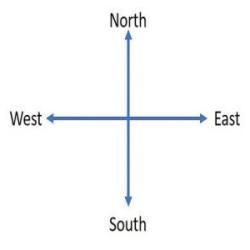Here, Batman is standing to left of Electro.

1. Antman, Batman, Cyclops, Deadpool, Electro, Falcon, and Gambit are standing near a wall and all of them are facing east.
2. Cyclops is on the immediate right of Deadpool.
3. Batman is at an extreme end and has Electro as his neighbor.
4. Gambit is between Electro and Falcon.
5. Deadpool is standing third from the south end.

Which of the following pairs are standing at extreme ends

a. Antman-Batman
b. Antman-Electro
c. Cyclops-Batman
d. Falcon-Batman
 Answer  Explanation ANSWER: Antman-Batman Explanation: Since all of them are facing East, the left and right should be taken into consideration accordingly.When a person is facing east, ‘Person’s Right’ will be towards South and ‘Person’s Left’ will be towards North.Cyclops is to the right of Deadpool.Deadpool is the third from south.So, Batman will be at extreme end from the north because it should have Electro as neighbor.Gambit is between Electro and Falcon.So, the sequence is -BatmanElectroGambitFalconDeadpoolCyclopsAntmanHere, Antman and Batman are at the extreme ends.

8)   4, 10, 28, ?, 244, 730

a. 24
b. 77
c. 82
d. 98
 Answer  Explanation ANSWER: 82 Explanation: In this series, each number is 2 less than 3 times the preceding number.The missing number : (28 x 3) – 2 = 82

9)   1, 11, 34, 77, 143, ?

a. 233
b. 235
c. 236
d. 237
 Answer  Explanation ANSWER: 237 Explanation: The given series is a triangular pattern series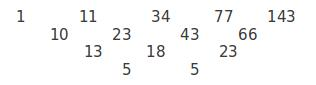The next term should be – 143 + (66 + 23 + 5 ) = 143 + 94 = 237

10)   In the question below is given a statement followed by two courses of action numbered I and II. You have to assume everything in the statement to be true and based on the information given in the statement, decide which of the suggested courses of action logically follow(s) for pursuing.

(A) If only I follows
(B) If only II follows
(C) If either I or II follows
(D) If neither I nor II follow
(E) If both I and II follow.

Statement - NGOs will not be provided any grant from Central Government.

Courses of Action - I. All the NGOs should be closed.

II. They should explore other sources of financial support.

a. Only I follows
b. Only II follows
c. Either I or II follows
d. Neither I nor II follow
e. Both I and II follow
 Answer  Explanation ANSWER: Only II follows Explanation: Closing organisations suddenly is not the solution to the problem.The NGOs must explore other sources to raise financial support. Thus, only II follows.

11)   In the question below is given a statement followed by two courses of action numbered I and II. You have to assume everything in the statement to be true and based on the information given in the statement, decide which of the suggested courses of action logically follow(s) for pursuing.

(A) If only I follows
(B) If only II follows
(C) If either I or II follows
(D) If neither I nor II follow
(E) If both I and II follow.

Statement - Five terrorists were caught with illegal explosives in the city.

Courses of Action - I. The Police should be on high alert to avoid any untoward incident.

II. The five terrorists should be set free and their movements should be watched to infiltrate the terror syndicate.

a. Only I follows
b. Only II follows
c. Either I or II follows
d. Neither I nor II follow
e. Both I and II follow
 Answer  Explanation ANSWER: Only I follows Explanation: There is no chance of releasing the criminals caught, they should be interrogated for information on other terrorists.Police should be on high alert and made cautious to prevent any untoward incident. So, only I follows.

12)   In the question below is given a statement followed by two conclusions numbered I and II.

(A) If only I follows
(B) If only II follows
(C) If either I or II follows
(D) If neither I nor II follows
(E) If both I and II follow.

Statement - Vipin was humiliated by the teacher in presence of his classmates.

Conclusions - I. The teacher disliked Vipin.

II. Vipin was not a mischievous boy.

a. Only I follows
b. Only II follows
c. Either I or II follows
d. Neither I nor II follows
e. Both I and II follow
 Answer  Explanation ANSWER: Neither I nor II follows Explanation: The teacher may have humiliated Vipin not because of his dislike but because of certain mistake.This does not make him mischievous. So, Neither I nor II follows.

13)   In the question below is given a statement followed by two conclusions numbered I and II.

(A) If only I follows
(B) If only II follows
(C) If either I or II follows
(D) If neither I nor II follows
(E) If both I and II follow.

Statement - Each man makes his own world; world is neither black nor white.

Conclusions - I. This world is black for some people.

II. This world is white for some people.

a. Only I follows
b. Only II follows
c. Either I or II follows
d. Neither I nor II follows
e. Both I and II follow
 Answer  Explanation ANSWER: Both I and II follow Explanation: The given statement says that each man manufactures a world for himself.Everyone has a different opinion about their life, so in this case, both I and II follow.

• Lalu Yadav began the rally from his house and went 150 km to the North.
• He turned west and covered 100 km.
• Then he turned south and covered 50 km.
• Finally turning to the east, he covered 100 km.
In which direction is he from his house?

a. East
b. West
c. North
d. South
 Answer  Explanation ANSWER: North Explanation: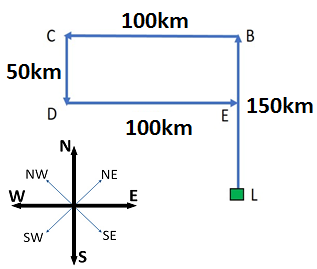• David put a timepiece on the table.
• He put it in such a way that at 6 P.M. hour hand pointed to North.
In which direction, the hour hand will point at 9 P.M.?

a. West
b. East
c. South
d. North
 Answer  Explanation ANSWER: East Explanation: At 9 P.M., the minute hand will point towards east.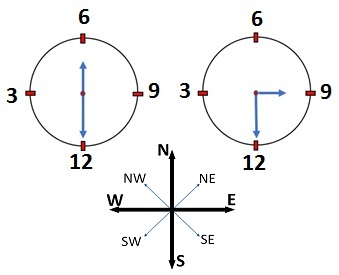16)   If Karan says, “Rocky’s mother is the only daughter of my mother”, How is Karan related to Rocky?

a. Brother
b. Father
c. Uncle
d. Grandfather
 Answer  Explanation ANSWER: Uncle Explanation: Daughter of Karan’s mother – Karan’s Sister.So, Karan is Rocky’s uncle.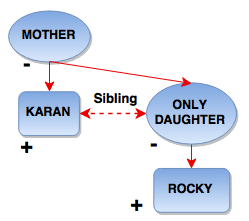17)   Ashwin introduces Rohit as the son of the only brother of his father’s wife.

How is Ashwin’s mother related to Rohit?

a. Cousin
b. Aunt
c. Uncle
d. Sister
 Answer  Explanation ANSWER: Aunt Explanation: Father’s wife – MotherMother’s brother – UncleUncle’s son – CousinSo, Ashwin’s mother is Aunt of Rohit.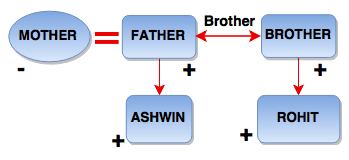1. Antman, Batman, Cyclops, Deadpool, Electro, Falcon, and Gambit are standing near a wall and all of them are facing east.
2. Cyclops is on the immediate right of Deadpool.
3. Batman is at an extreme end and has Electro as his neighbor.
4. Gambit is between Electro and Falcon.
5. Deadpool is standing third from the south end.

Who is standing exactly in the middle?

a. Electro
b. Falcon
c. Gambit
 Answer  Explanation ANSWER: Falcon Explanation: Since all of them are facing East, the left and right should be taken into consideration accordingly.When a person is facing east, ‘Person’s Right’ will be towards South and ‘Person’s Left’ will be towards North.Cyclops is to the right of Deadpool.Deadpool is the third from south.So, Batman will be at extreme end from the north because it should have Electro as neighbor.Gambit is between Electro and Falcon.So, the sequence is -BatmanElectroGambitFalconDeadpoolCyclopsAntman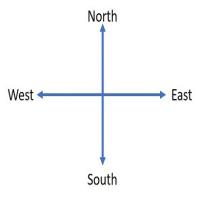Falcon is standing in the middle.

19)   1, 4, 27, 16, 125, ?, 343

a. 81
b. 64
c. 36
d. 25
 Answer  Explanation ANSWER: 36 Explanation: The given series consists of cubes of odd numbers and squares of even numbers i.e. 13, 22, 33, 42,…The next term is 62 = 36

20)   An accurate clock shows 10 o'clock in the morning. Through how many degrees will the hour hand rotate when the clock shows 6 o'clock in the evening?

a. 180
b. 190
c. 220
d. 240
 Answer  Explanation ANSWER: 240 Explanation: Hour hand travels 30 degree per hour.For, 8 hours, 8 x 30 = 240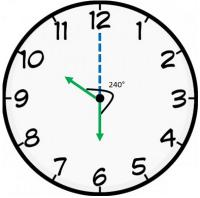21)   In the question below is given a statement followed by two conclusions numbered I and II.

(A) If only I follows
(B) If only II follows
(C) If either I or II follows
(D) If neither I nor II follows
(E) If both I and II follow.

Statement - Fortune favours the bold.

Conclusions - I. The necessary ingredient for success is risk.

II. Cowards never achieve success.

a. Only I follows
b. Only II follows
c. Either I or II follows
d. Neither I nor II follows
e. Both I and II follow
 Answer  Explanation ANSWER: Only I follows Explanation: According to the given statement, only those who face difficult situations bravely achieve success. So, I follows. II does not say anything related to the given statement, so, II does not follow.